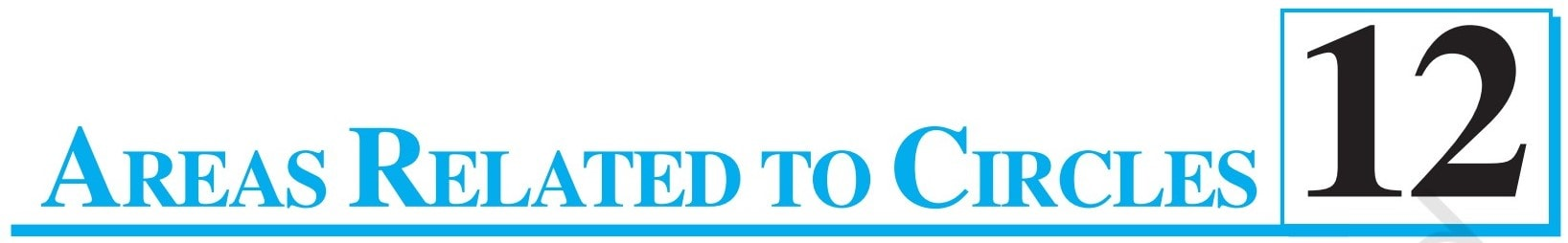# NCERT Solutions for Class 10 Maths

Ashish Kumar Let’s Learn is Online Learning Platform that provides classes through expert created online video lectures for Class 10 Maths and helps you build your basics, skills and advance your knowledge.

Here you can explore NCERT Solutions for Class 10 Maths with solutions of all questions given, with examples, important questions, supplementary exercise questions and Sample Papers through video lectures, pdf notes and assignments.

With the help of this digital platform and Virtually anyone with an internet connection and a commitment to self-indulgence can benefits this perfect opportunity to prepare for there CBSE Board exams.

## Select ChapterEuclid’s division lemma, Fundamental Theorem of Arithmetic – statements after reviewing work done earlier and after illustrating and motivating through examples. Proofs of results – irrationality of √2, √3, √5 , decimal expansions of rational numbers in terms of terminating/non-terminating recurring decimals.Zeros of a polynomial. Relationship between zeros and coefficients of a polynomial with particular reference to quadratic polynomials. Statement and simple problems on division algorithm for polynomials with real coefficients.Pair of linear equations in two variables. Geometric representation of different possibilities of solutions/inconsistency. Algebraic conditions for number of solutions. Solution of pair of linear equations in two variables algebraically – by substitution, by elimination and by cross multiplication. Simple situational problems must be included. Simple problems on equations reducible to linear equations may be included.Standard form of a quadratic equation ax^2 + bx + c = 0, (a ≠ 0) . Solution of quadratic equations
(only real roots) by factorization and by completing the square, i.e., by using quadratic formula. Relationship between discriminant and nature of roots. Problems related to day-to-day activities to be incorporated.Motivation for studying AP. Derivation of standard results of finding the nth term and sum of first n terms.Definitions, examples, counterexamples of similar triangles.
1. (Prove) If a line is drawn parallel to one side of a triangle to intersect the other two sides in distinct points, the other two sides are divided in the same ratio.
2. (Motivate) If a line divides two sides of a triangle in the same ratio, the line is parallel to the third side.
3. (Motivate) If in two triangles, the corresponding angles are equal, their corresponding sides are proportional and the triangles are similar.
4. (Motivate) If the corresponding sides of two triangles are proportional, their corresponding angles are equal and the two triangles are similar.

5. (Motivate) If one angle of a triangle is equal to one angle of another triangle and the sides including these angles are proportional, the two triangles are similar.
6. (Motivate) If a perpendicular is drawn from the vertex of the right angle to the hypotenuse, the triangles on each side of the perpendicular are similar to the whole triangle and to each other.
7. (Prove) The ratio of the areas of two similar triangles is equal to the ratio of the squares on their corresponding sides.
8. (Prove) In a right triangle, the square on the hypotenuse is equal to the sum of the squares on the other two sides.
9. (Prove) In a triangle, if the square on one side is equal to sum of the squares on the other two sides, the angles opposite to the first side is a right triangle.Review the concepts of coordinate geometry done earlier including graphs of linear equations. Awareness of geometrical representation of quadratic polynomials. Distance between two points and section formula (internal). Area of a triangle.Trigonometric ratios of an acute angle of a right-angled triangle. Proof of their existence (well defined); motivate the ratios, whichever are defined at 0° and 90°. Values (with proofs) of the trigonometric ratios of 30°, 45° and 60°. Relationships between the ratios.
Trigonometric Identities: Proof and applications of the identity sin^{2}A+cos^{2}A=1 . Only simple identities to be given. Trigonometric ratios of complementary angles.Simple and believable problems on heights and distances. Problems should not involve more than two right triangles. Angles of elevation/depression should be only 30​, 45 and 60.Tangents to a circle motivated by chords drawn from points coming closer and closer to the point.
1. (Prove) The tangent at any point of a circle is perpendicular to the radius through the point of contact.
2. (Prove) The lengths of tangents drawn from an external point to a circle are equal.Motivate the area of a circle; area of sectors and segments of a circle. Problems based on areas and perimeter / circumference of the above said plane figures. (In calculating area of segment of a circle, problems should be restricted to central angle of 60°, 90° and 120° only. Plane figures involving triangles, simple quadrilaterals and circle should be taken.)